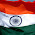# Gate Questions on Regular Expressions

(GATE-96)
Q1. Which two of the following four regular expressions are equivalent? (ε is the empty string).
(i) (00)*(ε + 0)
(ii) (00)*
(iii) 0*
(iv) 0(00)*
(a) (i) & (ii)      (b) (ii) & (iii)      (c) (i) & (iii)      (d) (iii) & (iv)

Sol: Option (b) is correct.
Explanation:
i. (00)*( ε + 0) = (00)*ε + (00)*0 = (00)* + (00)*0 = 0*
Note that (00)* Generates strings of even length and (00)*0 generated the strings of odd length. Since + operator Union of both sets results in 0*.

(GATE-98)
Q2. The string 1101 does not belong the set represented by
(a) 110*(0+1)
(b) 1(0+1)*101
(c) (10)*(01)*(00+11)*
(d) (00+(11)*0)*

Sol:
Both option (c) and (d) will not generate the string 1101

(GATE-98)
Q3. if the regular set A is represented by A = (01+1)* an the regular set B is represented by B = ((01)*1*)*, which of the following is true?
(a) A Ì B
(b) B Ì A
(c) A and B are incomparable
(d) A = B

Sol: Option d is true.
Explanation:
A = (01+1)*
A = ( (01)* 1* )*       -- from rule (r1 + r2)* =( r1*r2*)*
A = B

Note:
If r1 and r2 are two regular expressions then,
(r1 + r2)* = (r1* + r2*)*
= (r1* + r2)*
= (r1 + r2*)*
= (r1* . r2*)*

(GATE-2000)
Q4. Let S and T be languages over ∑ = {a,b} represented by the regular expression (a + b*)* and (a + b)*, respectively. Which of the following is true?
(a) S Ì T
(b) T Ì S
(c) S = T
(d) S È T = f

Sol:Option c is true.
Explanation:
From rule (r1 + r2)* =( r1 + r2*)*.
Note: Both S and T will generate the same language.

1.1.